# Iterated Functions Systems for a Disk

## in the Fractal Gallery of William Gilbert

Pure Mathematics Department, University of Waterloo, Ontario, Canada
These are all Iterated Functions Systems (IFS) using two maps on the polar form of complex numbers.

## Pictures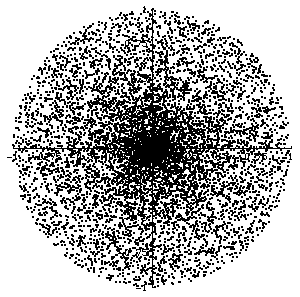DiskIFS0
IFS for a disk

using two maps applied to the polar form (r, a) of complex numbers:
Fi(r, a) = (a/(2Pi), (r + i)Pi)
where i = 0 or 1 and 0 =< r =< 1 and 0 =< a =< 2Pi.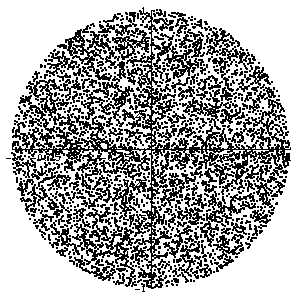DiskIFS
IFS for a disk with constant density

Define an IFS in the (r,s)-plane, consisting of two linear maps, who attractor is the triangle
0 =< r =< 1 and 0 =< s =< r.
Then map the point (r,s) in the triangle to the point in the disk with polar form (r, a) where
a = 2(s/r)Pi.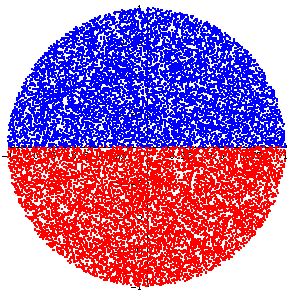DiskIFS2
IFS for a disk with constant density
showing the images of the two maps

The maps are the same as in the previous example.
The colors represent the images of the two maps.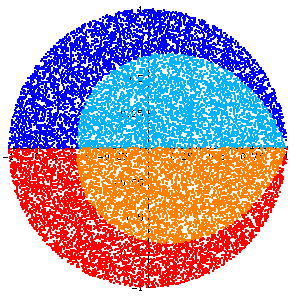DiskIFS4
IFS for a disk with constant density
showing the images after two iterates

The maps are the same as in the previous two examples.
The colors represent the four possible images after two iterates of the two maps.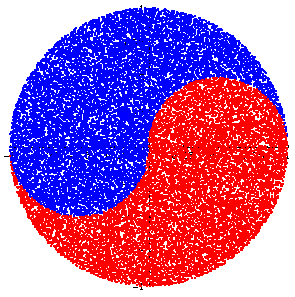YinYangIFS
Yin Yang IFS

A modification of the maps of the previous examples, showing the images of the two maps.
The point (r,s) in the triangle gets mapped to the point in the disk with polar form (r, a) where
a = 2(s/r)Pi + ArcCos(r).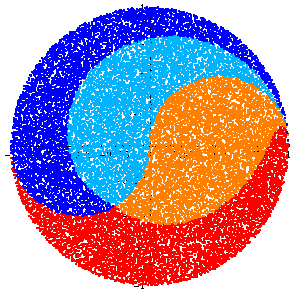YinYangIFS4
Yin Yang IFS

The maps are the same as in the previous example.
The colors represent the four possible images after two iterates of the two maps.

© 2002 by William Gilbert          Back to the entrance of the Fractal Gallery.